# Algebra II : Graphing Circle Functions

## Example Questions

### Example Question #1 : Graphing Circle Functions

The graph of the equation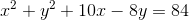is a circle with what radius?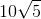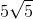Explanation:

Rewrite the equation of the circle in standard form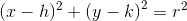as follows: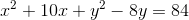Since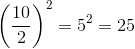and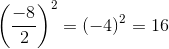, we complete the squares by adding: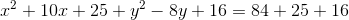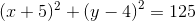The standard form of the equation sets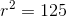,

so the radius of the circle is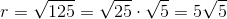### Example Question #2 : Graphing Circle Functions

Determine the graph of the equation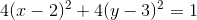Circle, centered at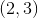with radius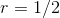Ellipse, centered atHyperbola, centered atCircle, centered atwith radius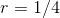Circle, centered atwith radiusExplanation:

The equation of a circle in standard for is: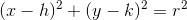Where the center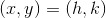and the radius of the cirlce is.

Dividing by 4 on both sides of the equation yields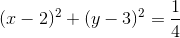or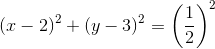an equation whose graph is a circle, centered at (2,3) with radius = .5

### Example Question #64 : Quadratic Functions

Give the radius and the center of the circle for the equation below.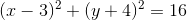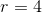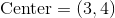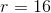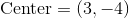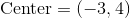Explanation:

Look at the formula for the equation of a circle below.Here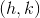is the center andis the radius. Notice that the subtraction in the center is part of the formula. Thus, looking at our equation it is clear that the center is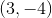and the radius squared is. When we square root this value we get that the radius must be### Example Question #1 : Graphing Circle Functions

Determine the equation of a circle whose center lies at the point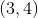and has a radius of.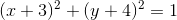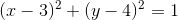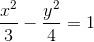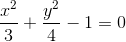Explanation:

The equation for a circle with centerand radiusis :Our circle is centered atwith radius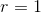, so the equation for this circle is :### Example Question #66 : Quadratic Functions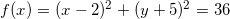What is the radius of the circle?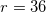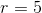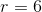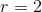Explanation:

The parent equation of a circle is represented by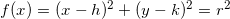. The radius of the circle is equal to. The radius of the cirle is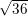.

### Example Question #67 : Quadratic Functions

What is the center of the circle expressed by the funciton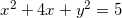?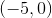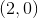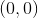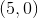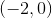The equation can be rewritten so that it looks like the parent equation for a circle. After completeing the square, the equation changes fromto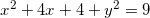. From there it can be expressed as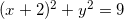. Therefore the center of the circle is at.